The relation between the graphs structures and proof complexity of corresponding Tseitin graph tautologies

Armen Mnatsakanyan

Yerevan State University,Department of Informatics and Applied Mathematics, Armenia

E-mail: arm.mnats@gmail.com

# Abstract

We describe sufficient properties of graphs Gn on n vertices such that the formulas constructed by Tseitin’s method on them require exponential Resolution proof steps. The square grid style graphs of Tseitin formulas and expanded graphs of Urquhart are examples of graphs with mentioned properties. If these properties are not valid for any graph, then the corresponding formula has polynomial bounded resolution refutation.

Keywords: graph based formula, graph structure, proof complexity.

# 1. Introduction

There are many well-known examples of tautologies, which require exponential proof complexities in weak systems. Some of them are graph-based formulas introduced by Tseitin in . As Tseitin graph tautologies, constructed on the base of different graphs, have different proof complexities, it is interesting to investigate the relation between the structure of graphs and proof complexities of corresponding Tseitin graph tautologies. In  A.Urquhart constructed the sequence of graphs such that the formulas based on them are hard examples for Resolution.

We describe sufficient properties of graphs Gn on n vertices such that the formulas based on them require exponential Resolution proof steps. The square grid style graphs of Tseitins formulas and expander graphs of Urquhart are examples of graphs with mentioned properties. If properties are not valid for any graph, then the corresponding formula has polynomial bounded resolution refutation.

# 2. Main notions and notations

In this chapter we use the main notions and notations, which are introduced in [1,2] to describe Tseitin graphs and proof complexities of corresponding Tseitin graph tautologies in Resolution system. It is well known that Classical Resolution proves a formula to be a tautology by showing that its negation, which is put into conjunctive normal form, is unsatisfiable. Each propositional variable p has a conjugate (or negation) denoted by p. A literal is a propositional variable p or a conjugate p. A clause is a finite set of literals, where the meaning of the clause is the disjunction of the literals in the clause.

Resolution has no fixed axioms. It has only one inference rule called resolution rule (or annihilating rule): from clauses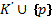and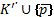follows clause. When we try to show that a set of clauses C is unsatisfiable, we take C to be a set of hypotheses, to which we apply the resolution rule until we obtain the empty clause ^. A proof of the empty clauses is a resolution refutation. If the proof is viewed as a tree, the complexity is the number of distinct clauses occurring in the tree.

Follower  by a graph we here mean a finite symmetric graph without loops. We will assume, in addition, that each edge of the graph is associated with a pair of conjugate variables (distinct pairs being associated with distinct edges).

If G is a graph, then a labeling G’ of G is an assignment of literals to the edges of G, so that distinct edges are assigned literals that are distinct and not complements of each other, together with an assignment Charge(x)Î{0,1} to each of the vertices x in G. The total charge Charge(G’) of G is the sum modulo 2 of the charges attached to the vertices of G.

If G’ is a labeled graph, and x a vertex in G’, and l1,…,lk the literals labeling the edges attached to x, then Clauses(x) is the set of clauses equivalent to the conjunctive normal form of the modulo 2 equation l1ÅÅlk = Charge(x). That is to say, a clause C is Clauses(x) contains the literals l1,…,lk, and the parity of the number of complemented literals in {l1,…,lk} in C is opposite to that of Charge(x). The set of clauses Clauses(G’) is the union of all the sets Clauses(x), for x a vertex in G. As |Clauses(G’)| we will denote number of clauses in Clauses(G’) .

It is known from  that the set Clause(G’) for a connected graph G is unsatisfiable if and only if Charge(G’)=1.

For x vertex of G, as d(x) we denote degree of vertex x: number of edges attached to x. As d(G) lets denote maximum vertex degree among all vertices.

Let us also remind the well-known following terminology related to graph structures. The cyclomatic number of graph G (denoted by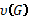) is the number of edges of the graph minus the number of vertices plus the number of connected components (i.e., the cyclomatic number is the rank of the one-dimensional group of cycles). Let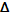be a partial subgroup of graph G. We will say that edge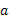of G emerges fromifdoes not belong to, but has at least one vertex in common with. Let G\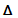denote the sub-graph of G obtained by the removal of all vertices of(together with all edges incident to these vertices). Let as takeIt can be easy seen that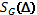is the maximum number of edges emerging from,which can be removed without disrupting the connectedness of G.

Based on  we will define proof complexityas minimum steps among all proofs of tautology for given graph G. Theorem 7 and Lemma 17 in  give following equation for proof complexity:whereis partial subgroup of graph G, andis the maximum number of edges emerging fromwhich can be removed without disrupting the connectedness of G. This statement shows that proof complexity depends only from given graph; therefore on graph structure we will have different proof complexities. In next chapter it will be described some relationship between graph structure and proof complexity.

# 3. Proof complexity dependence on graph structure

In this chapter it will be given main theorem about relation between the graph structures and proof complexity of corresponding Tseitin graph tautologies. For connected graph Gn with n vertices following lemma is true:

Lemma 1.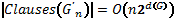Proof. We know that: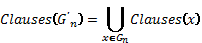As all clauses assigned to all vertices are distinct, we will have: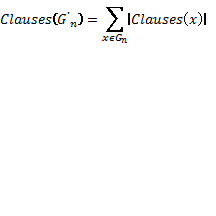Clauses(x) is the set of clauses equivalent to the conjunctive normal form of the modulo 2 equation l1ÅÅlk = Charge(x). This means that:And for |Clauses(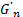)| we will have: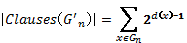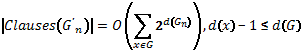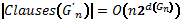¿

Main Theorem.

Proof.From previous chapter we know that: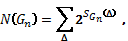where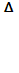is any sub-graph of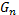, andis the maximum number of edges emerging fromthat can be removed without disrupting the connectedness of. As the maximum vertex degree is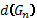, it is possible to remove maximum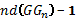edges not to lose connectivity betweenand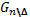: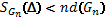As the total number of different sub-graphs is , we will have following result: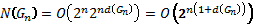¿

This theorem gives as information about hardiest examples that could be constructed with Tsaitin graphs. It says that among all graphs with maximum vertex count, proof complexity can be maximum . Lets look into following cases of Tsaitin graph structures:

1., it is not dependent on number of vertices n. For graphs with constant maximum vertex degree, we can create hardiest tautologies with maximum proof complexity equal .Based on Lemma 1 number of clauses are less than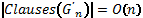2.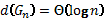. Maximum possible proof complexity is .And number of clauses are less than  nclauses: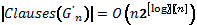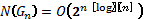3.: As above we have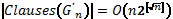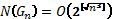4.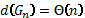: By analogy we have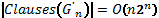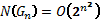This case represents graph with maximum vertex degree equal to number of vertices (full graph is one example). These types of Tseitin tautologies are not considered hard because number of clauses is about the same as proof complexity.

These 4 cases gives clear vision about dependence of graph structure and proof complexity of its Tsetin tautology. The square grid style graphs of Tseitins formulas and expander graphs of Urquhart are examples of case 1, they havelimited by some constant exponential proof complexity. In next chapter it will be constructed hardiest possible examples for each described case.

# 4. Hardiest Tseitin graph examples

In previous chapter it was introduced 4 common types of graph structures and proof complexities associated with them. We haveupper boundfor each complexity. For each case we will create hardiest possible examples with proof complexity. Here are 4 examples for each case:

Case 1:. It is possible to create graph with proof complexity equal to. Here is example of graph with n vertices in circular style; each vertex has common edge with its neighbors and neighbors of their neighbors, like in picture below.000251659264251658240

In first chapter there was described following equation for proof complexity:Whereis any sub-graph of, and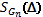is the maximum number of edges emerging fromthat can be removed without disrupting the connectedness of. In our case it is obvious that it for each sub-graphthere are more than one connecting edges betweenand. And it is possible to remove one edge not to lose connectivity: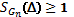. This means: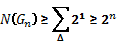From previous chapter we had N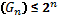equation for this case. So: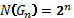Case 2:It is possible to enhance first example to have maximum vertex degree aboutlog(n). Suppose we have circular graph with n vertices as described in case 1. Lets number vertices of graph G starting from 1 to n in clockwise order. Vertex i and j vertices will be connected with edge if distance between them is power of two. In formal way i is connected to j if: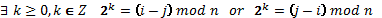It is obvious that each graph vertex will have degree with log(n) complexity. In this case for each sub-graph there will be log(n) edges which can be removed not to lose connectivity and proof complexity for corresponding Tseitin tautology will be: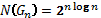Case 3. For circular graph we can connect i and j if following statement is true:In similar way we will have following proof complexity: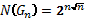Case 4. Simplest graph for this case is full graph with n vertices; it has max vertex degree equal to n and following proof complexity: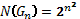Proof. Suppose sub-graphof full graph has k vertices, there are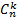such sub-graphs. Each vertex has connection with each n-k vertices ofsub-graph. There are total k*(n-k) connecting edges betweenand. Asandare full graphs as well it is necessary to keep only one edge not to lose connectedness. This means that we can remove (k*(n-k)-1) edges by keeping connectedness:For proof complexity we will have: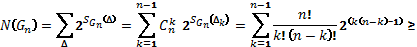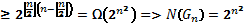Summarizing this 4 examples it is possible to create universal hardiest graph for any possible value of. It is needed to construct circular graph and connect i and j vertices with following rule: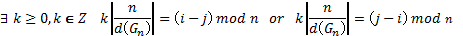And it will have following proof complexity: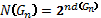Acknowledgment. Grant 13-1B004 of SSC of Government of RA supports this work. Thanks to my supervisor Anahit Chubaryan for valuable comments, corrections and suggestions.

# References

1.   G.S.Tseitin, on the complexity of derivation in propositional calculus, Studies in   constructive mathematics and mathematical logic, part 2, New York, 1970, pp.115-125.

2.   A.Urquhart, Hard examples for resolution, Journal of the Association for Computing Machinery, vol. 34 (1987), pp. 209-219.